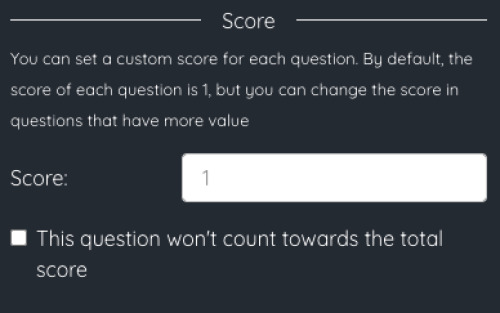How to set a custom score for each question

By default, each question you add to the worksheet will have the same weight. If you do not change it, the default value will be 1.

You can change the score of each question, so that questions that have more value count more towards the final grade. For example, if you set the score of a question as 2, that question will score double than the other questions when calculating the final grade.

The calculation of the final grade will be done by adding the value of all the questions answered correctly and normalizing the grade over 10.For questions of type Answer and Selector, there is an option to not count the question when calculating the grade. This will be useful, for example, to add fields where the student can write his name or a comment on the worksheet that should not be counted in the grade.